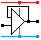Home

rOm
Quest
Glossary

Random
Page
Search
Site
Lush
Sim
Class
Subject
Images

Help
FAQ
Sign
Up
Log
In
 A Level     >Logic<     Tristate

# Logic

Site for Eduqas/WJEC - Go to the AQA site.

A Level    Tristate Logic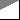Questions 0 to 0   -->  View All

Logic Gates are digital switching circuits that obey simple rules.
The inputs and outputs are LOW ( 0 ) or HIGH ( 1 ) and should never float halfway between.

A LOW signal is a voltage less than half the power supply, usually close to zero.
A HIGH signal
is a voltage more than half the power supply, usually close to five volts ( or 3.3V in low voltage logic ).
Never leave inputs unconnected. They float, act as an antenna, and pick up every noise signal in range.

• The Gate Symbols.
• The Boolean Notation.
• The Plain English Rules. - Especially learn the yellow highlights.
• The Truth Tables (Two Styles) - Especially learn the yellow highlights.
AND NAND OR NOR XOR XNOR NOT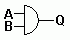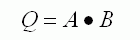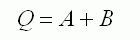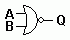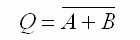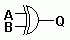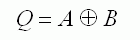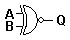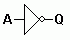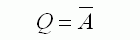Two ones give a one.
Anything else gives 0.
Two ones give a 0.
Anything else gives 1.
Two zeros give a 0.
Anything else gives 1.
Two zeros give a 1.
Anything else gives 0.
Equal inputs give a 0. Equal inputs give a 1. Input is inverted.
```A B   Q
0 0   0
0 1   0
1 0   0
1 1   1
```
```A B   Q
0 0   1
0 1   1
1 0   1
1 1   0
```
```A B   Q
0 0   0
0 1   1
1 0   1
1 1   1
```
```A B   Q
0 0   1
0 1   0
1 0   0
1 1   0
```
```A B   Q
0 0   0
0 1   1
1 0   1
1 1   0
```
```A B   Q
0 0   1
0 1   0
1 0   0
1 1   1
```
```A  Q
0  1
1  0

```
 B\A 0 1 0 0 0 1 0 1
 B\A 0 1 0 1 1 1 1 0
 B\A 0 1 0 0 1 1 1 1
 B\A 0 1 0 1 0 1 0 0
 B\A 0 1 0 0 1 1 1 0
 B\A 0 1 0 1 0 1 0 1
 A 0 1 Q 1 0

## Logic Levels

 CMOS 4000 Series Chips TTL 7400 Series Chips Commonly Used Terms Technology Complementary Metal Oxide Semiconductor Transistor Transistor Logic LOW A voltage less than 0.5 of the power supply 0 to 0.8 V 0, Zero, Off, Clear, False HIGH A voltage greater than 0.5 of the power supply 2.2 to 5 V 1, One, On, Set, True Power Extremely low power consumption High power use compared with CMOS Speed Slower Faster

## Boolean Algebra

BOOLE: A mathematician called Boole invented a branch of maths for processing true and false values instead of numbers. This is called Boolean Algebra. Simple Boolean algebra is consistent with common sense but if you need to process decisions involving many values that might be true or false according to complex rules, you need this branch of mathematics. Boolean algebra was invented long before the invention of logic gates!

## Logic Gate Rules

There are several types of gate. Each follows a very simple set of rules. By combining many gates in suitable ways, processing devices can be produced. A computer CPU chip can have millions of gates fabricated onto it. The table above shows several gates with two inputs. Many of these gates are also available in three, four and eight input versions.

Computers work using LOGIC. Displaying graphics such as the mouse cursor involves the XOR (Exclusive OR) operation. Arithmetic addition makes use of AND and XOR.

## Truth Tables

The one line descriptions of the rules above are clearer if shown in Truth Tables. These tables show the output for all possible input conditions. The inputs are always listed in the same order (counting in binary starting from zero).

EXAMPLE: There are two inputs, A and B. Input B is inverted by the NOT gate to give X.
If A and X are both high the AND gate output will go high and the alarm will sound.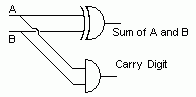This is one of the simplest logic circuits that does something useful. It adds two bits together.

```A      B   C  S
0 plus 0 = 0  0
0 plus 1 = 0  1
1 plus 0 = 0  1
1 plus 1 = 1  0
```
• The S or Sum column is identical to the XOR gate rule so this gate can be used.
• The C or Carry column stores the result of adding 1 plus 1 giving 10 (two in binary), the AND gate can be used to generate this output.
• By combining 32 or 64 full adders, you are part way to building your first CPU chip for a fully featured computer.

Subject Name     Level     Topic Name     Question Heading     First Name Last Name Class ID     User ID

 CloseEscapeX Q: qNum of last_q     Q ID: Question ID         Score: num correct/num attempts         Date Done

Question Text

image url

Help Text
Debug

• You can attempt a question as many times as you like.
• If you are logged in, your first attempt, each day, is logged.
• To improve your scores, come back on future days, log in and re-do the questions that caused you problems.
• In the grade book, you can delete your answers for a topic before re-doing the questions. Avoid deleting unless you intend re-doing the questions very soon.